﻿ Pythagorean theorem stock image. Image of square, chalkboard - 32125083

# Pythagorean theorem.

## Pythagorean theorem sketched with white chalk on a chalk board

Designers also selected these stock photos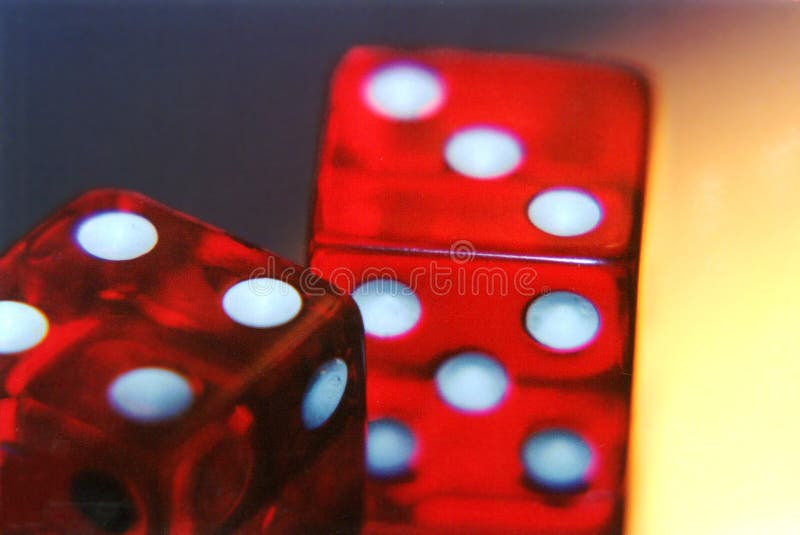DiceDaisyShoal anthias fish on the coral reef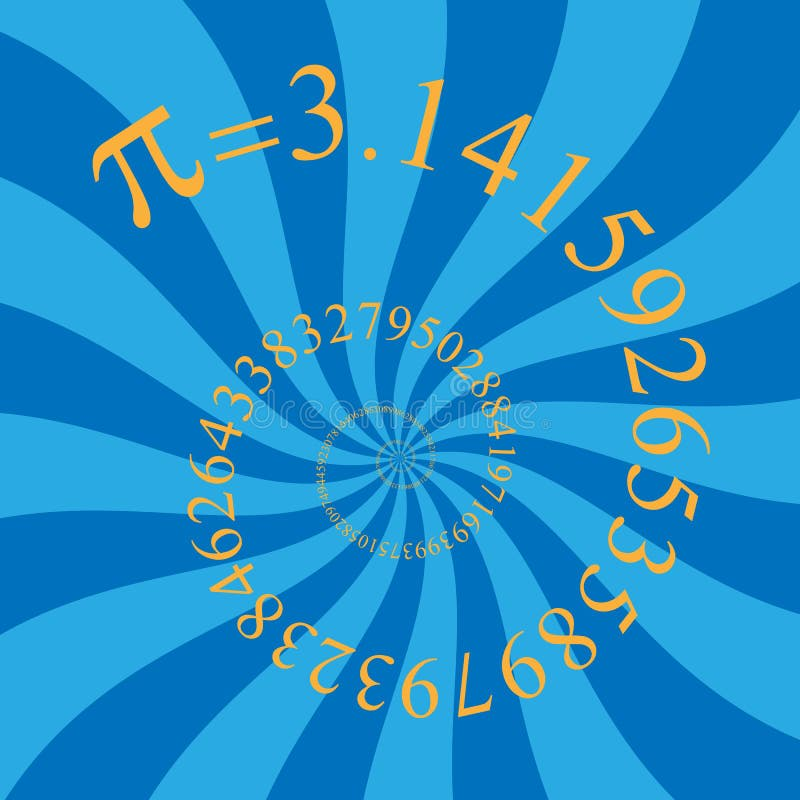PiClose up nautilus shell pattern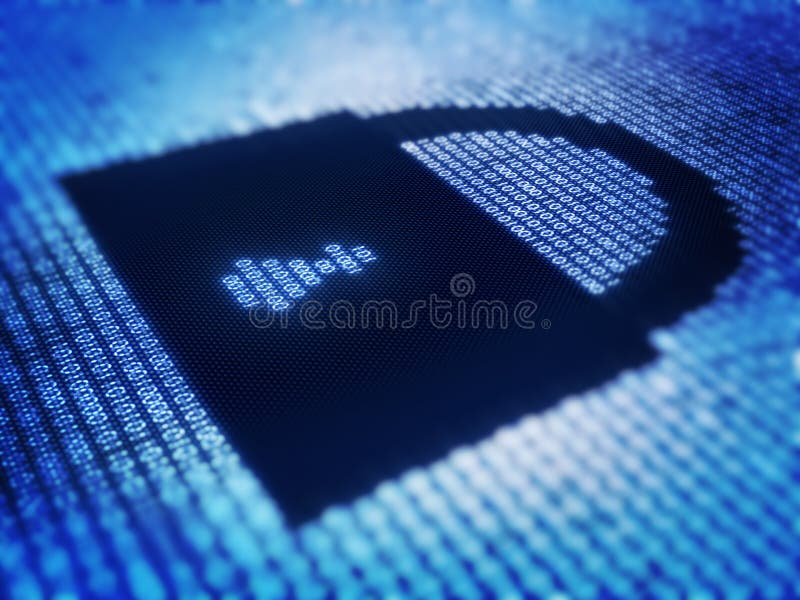Binary code and lock shape on pixellated screenRipe coconuts in market as background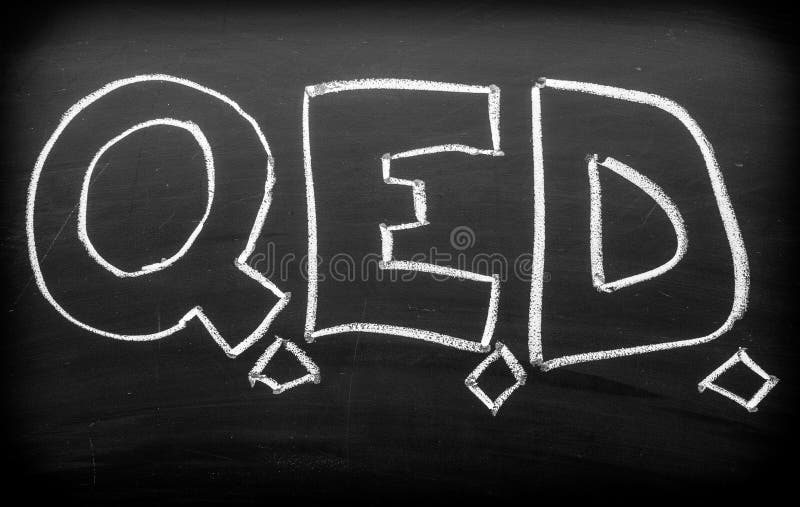QED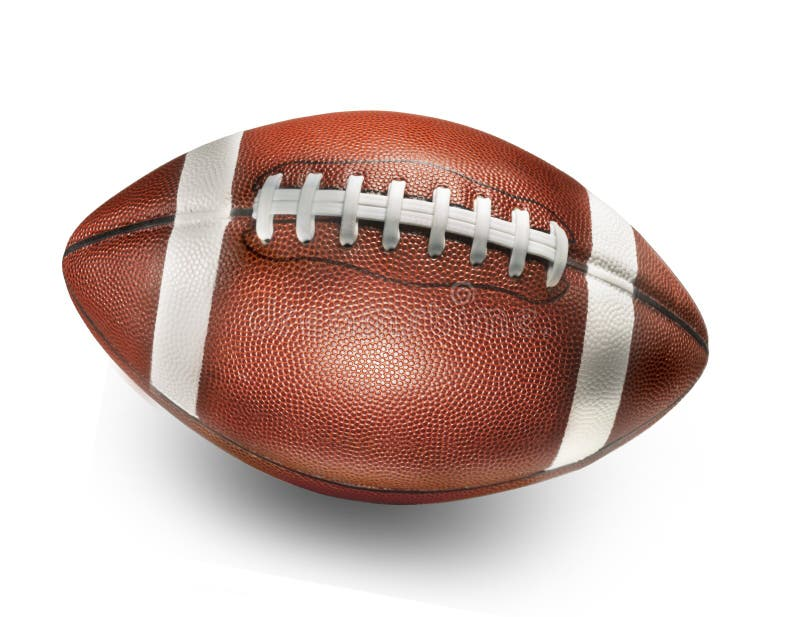Football
More similar stock images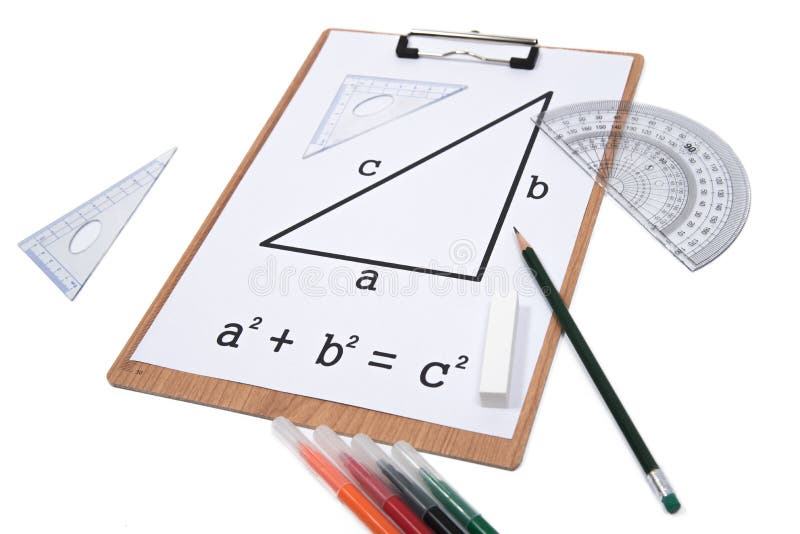Pythagorean Theorem.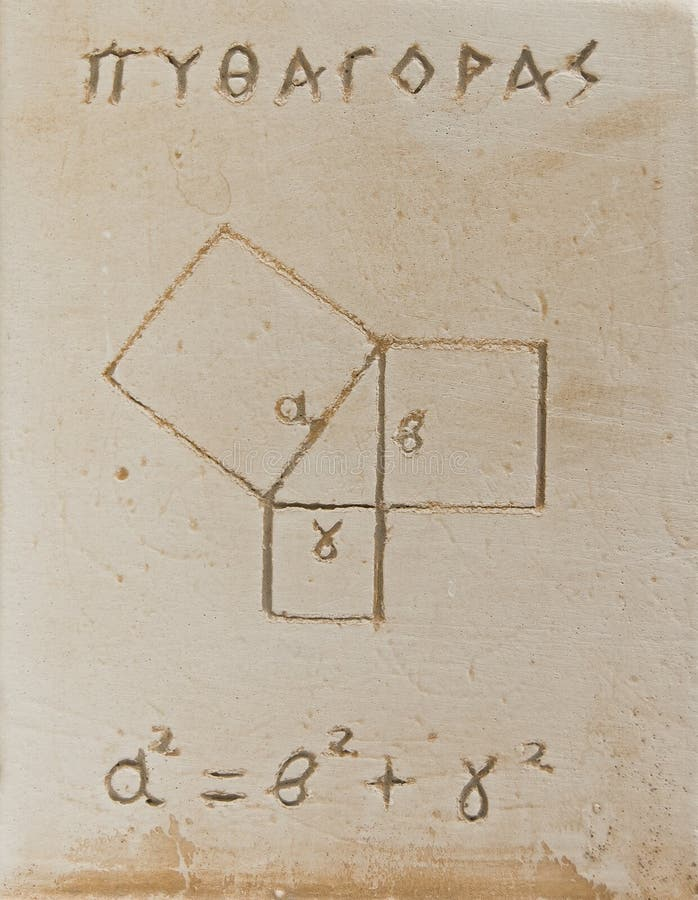Pythagorean theorem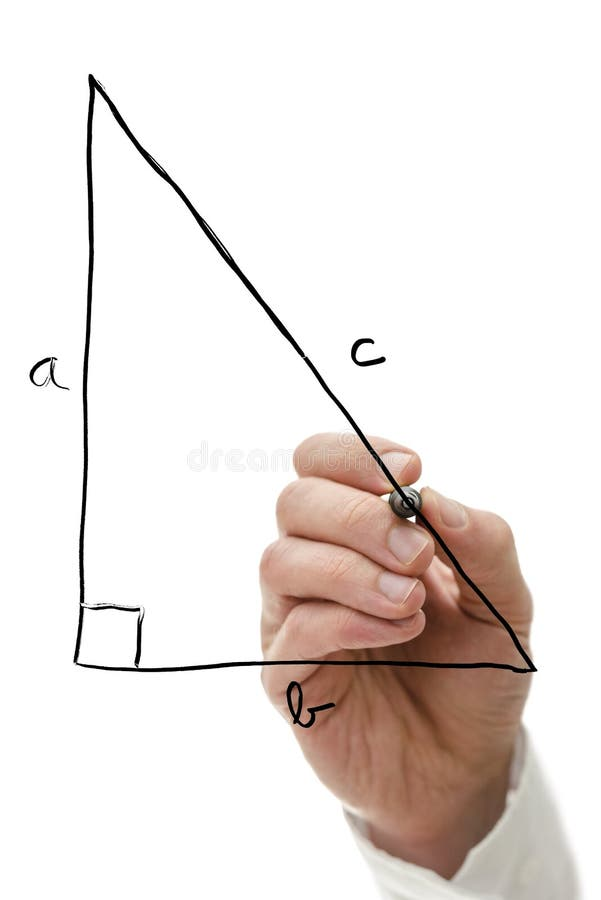Pythagorean theorem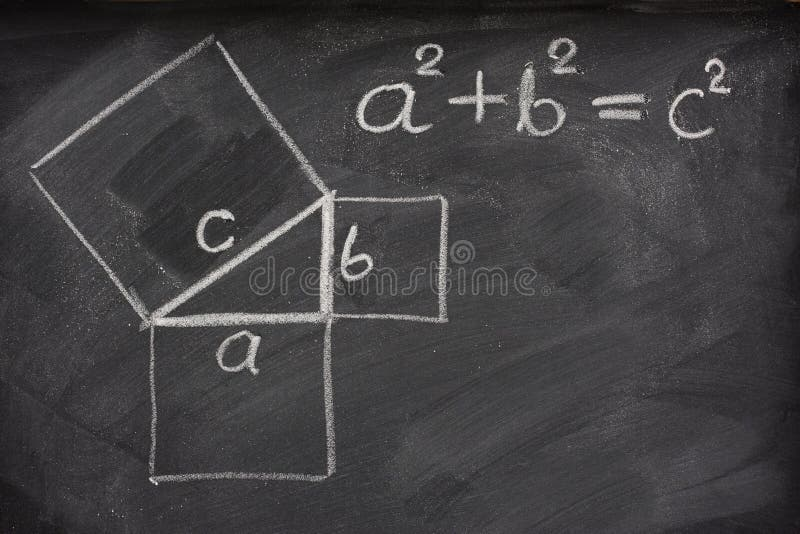Pythagorean theorem on blackboard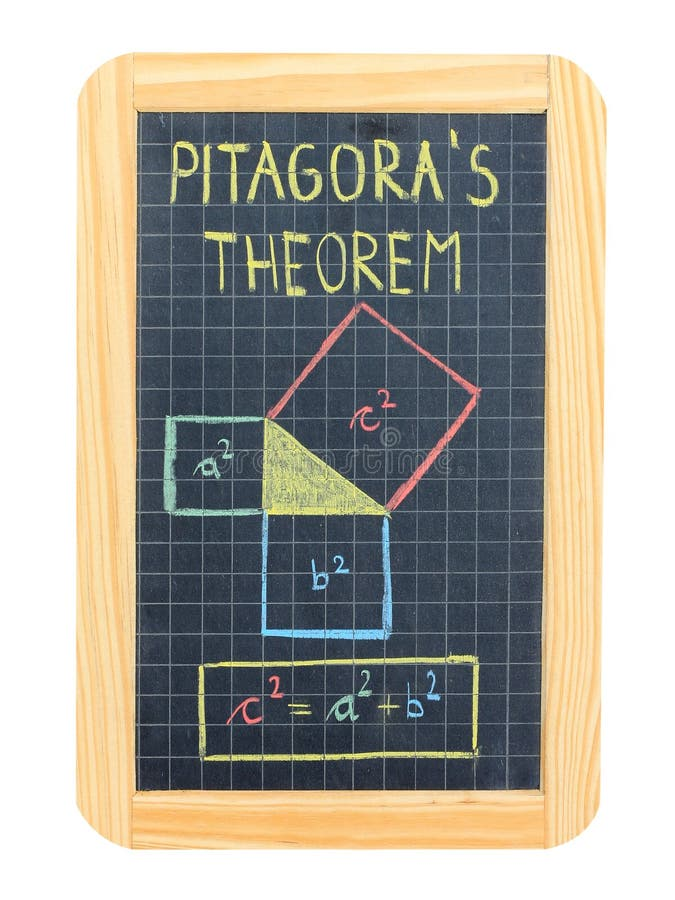Pythagorean theorem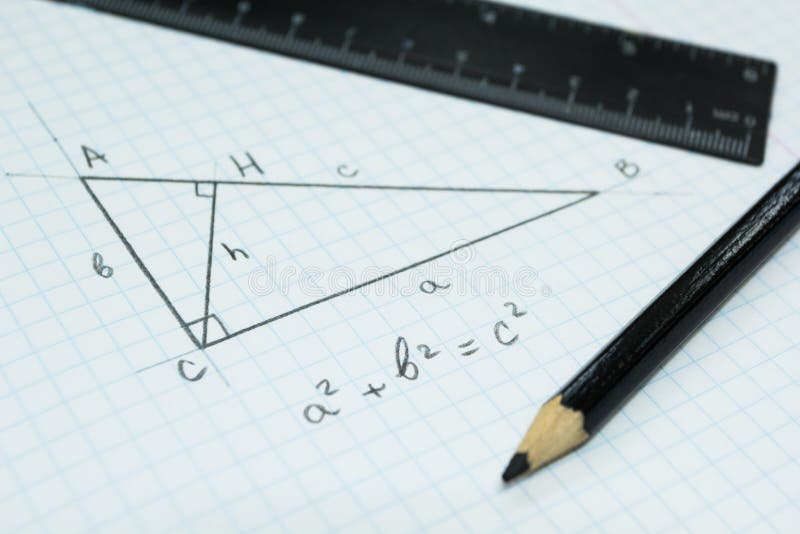Geometry, the Pythagorean theorem in a school notebook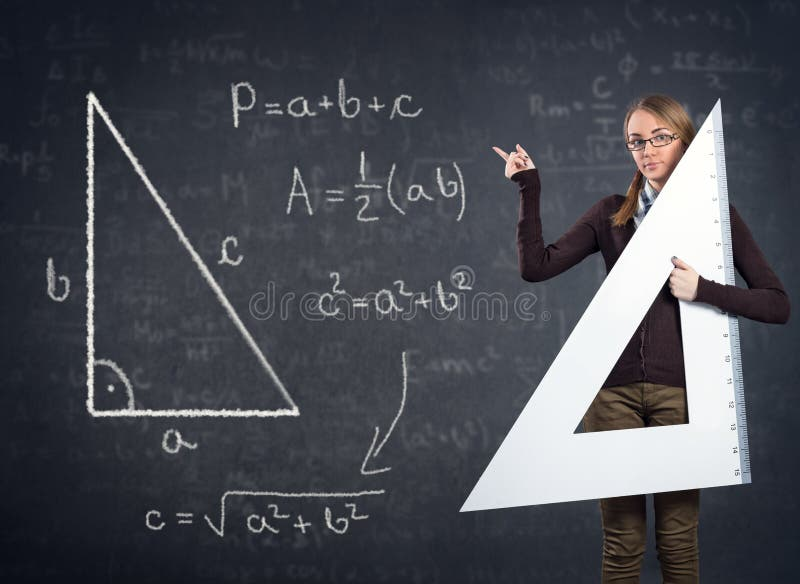Student with a big ruler and Pythagorean theorem on blackboard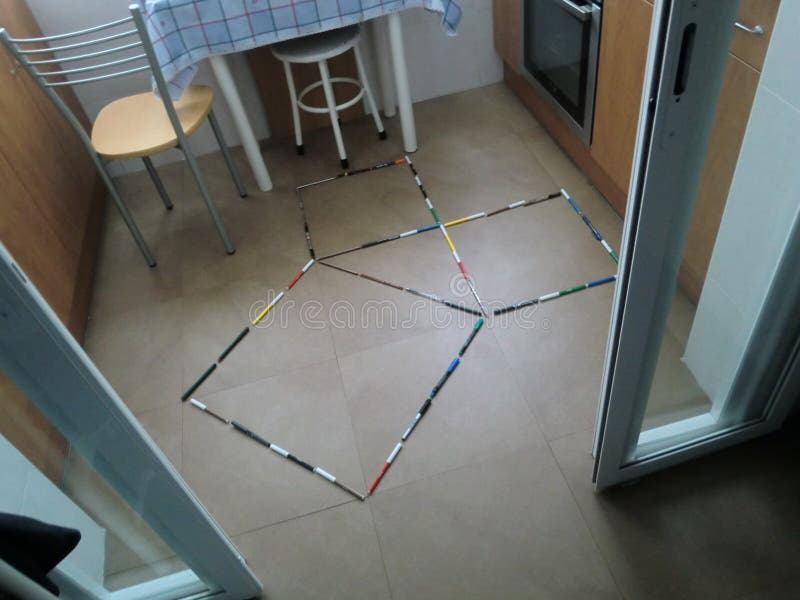Graphical demonstration of the Pythagorean Theorem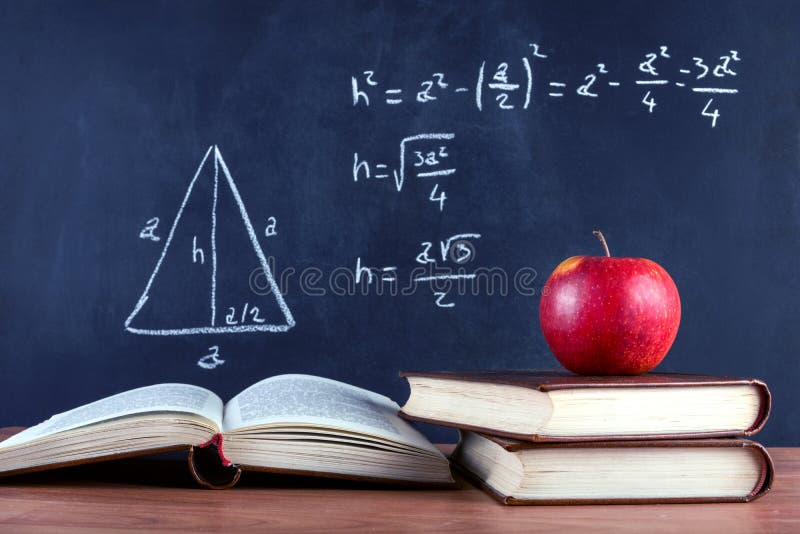Apple on books and blackboard with Pythagorean theorem
More stock photos from Igor Stevanovic's portfolio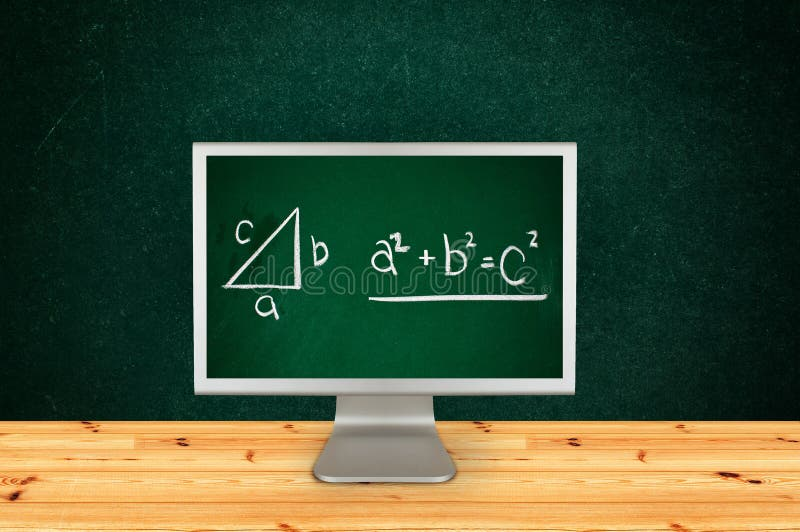Pythagorean theorem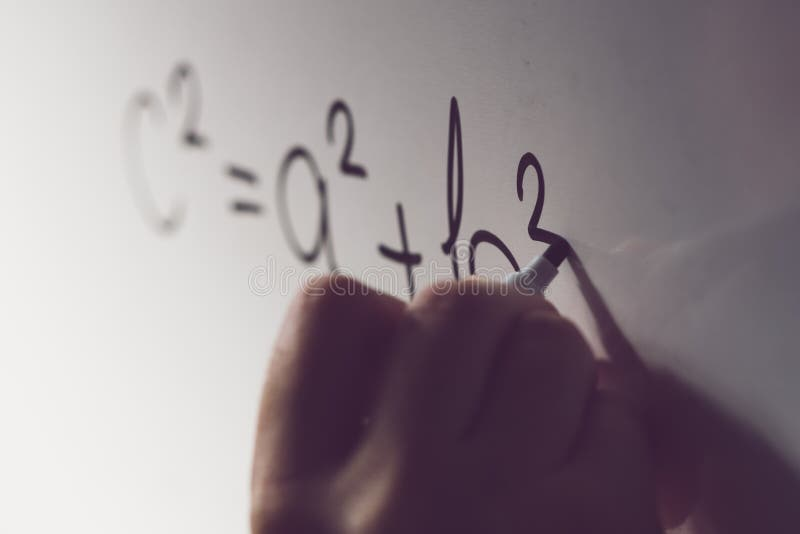Pythagorean theorem, math class in the school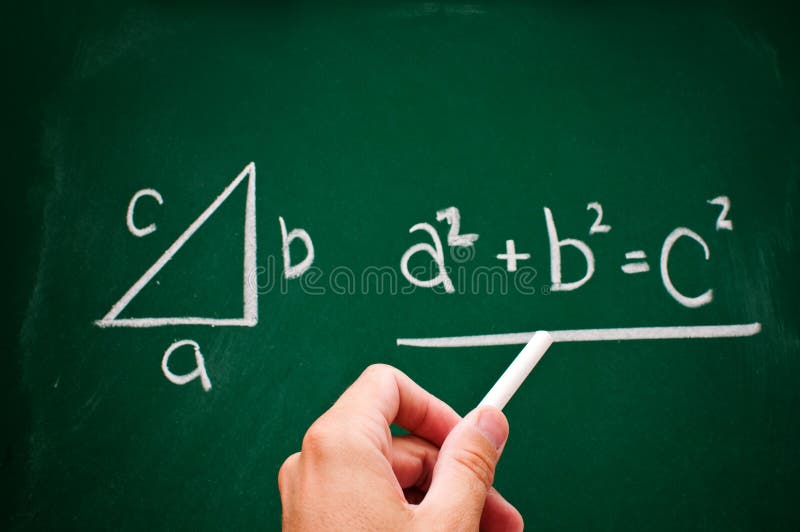Pythagorean theorem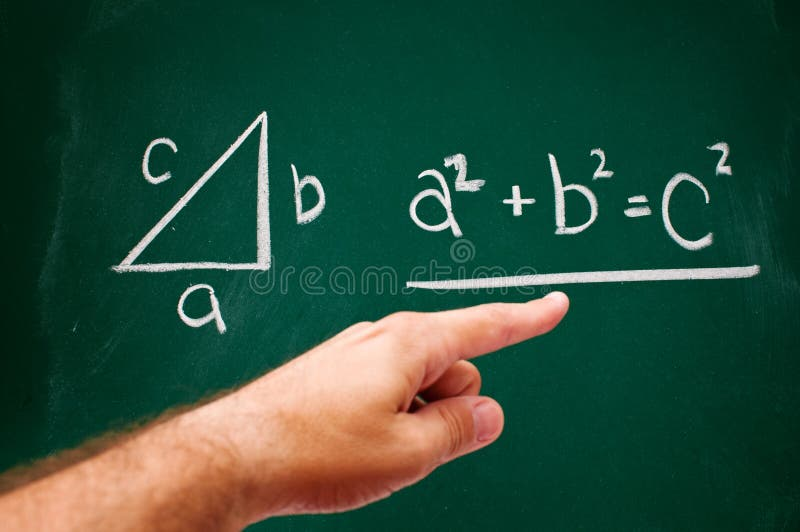Pythagorean theorem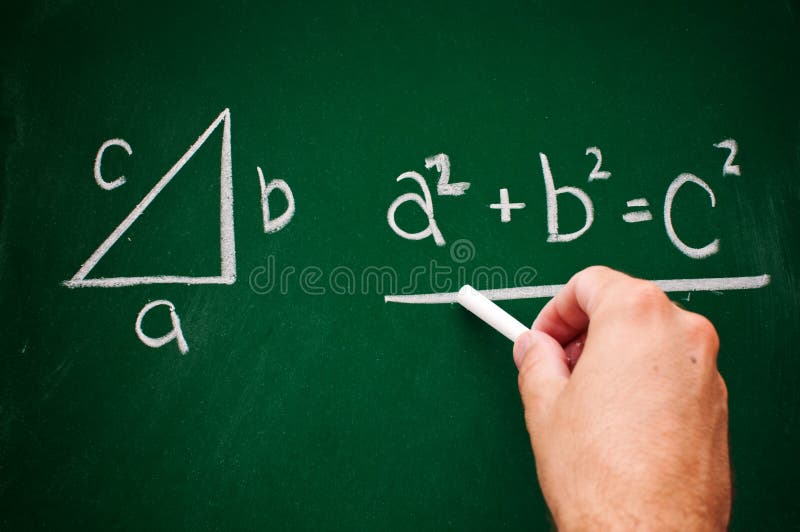Pythagorean theorem
Related categories